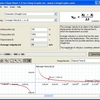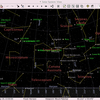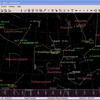# Gravity

How fast does gravity travel? What is it about mass that causes the force to exist? Is gravity a particle? Gravity has raised many unanswered questions throughout the ages. It is a force that we are all accustomed to in our daily lives - and yet how much of the subject do we really understand? This gravity calculating tool is designed to help determine the force that should exist between two objects containing mass.

## Related software (5)## Physics Cheat Sheet

Physics Cheat Sheet is an interactive physics package that helps students solve and visualize numerous physics equations.## Systems of Nonlinear Equations

Numerically solves systems of simultaneous nonlinear equations. It can fully explore defined intervals to search for multiple solutions or quickly find solutions starting with rand ...## Ordinary Differential Equations

Solves boundary-value problems involving ordinary differential equations producing a finite series valid in the entire defined interval. Handles a wide variety of functions, includ ...## AstroGrav for Mac

A full-featured, high precision solar system simulator that calculates the gravitational interactions between all astronomical bodies, so that the motions of asteroids and comets a ...## AstroGrav

A full-featured, high precision solar system simulator that calculates the gravitational interactions between all astronomical bodies, so that the motions of asteroids and comets a ...